Fingerings Home Octave 1: F  (C) Gb  (Db) G  (D) Ab  (Eb) A  (E) Bb  (F) B  (Gb) C  (G) Db  (Ab) D  (A) Eb  (Bb) E  (B) Octave 2+: F  (C) Gb  (Db) G  (D) Ab  (Eb) A  (E) Bb  (F) B  (Gb) C  (G) Db  (Ab) D  (A) Eb  (Bb) E  (B) F  (C) Gb  (Db) G  (D)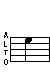E (B) - 1st Octave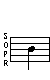## Trills

Trill - From Gb
(See below for alternate)
Trill - From F
(See below for alternate)
Gb (alt) E   F E (alt)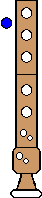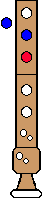Alternate Trill - From Gb   Alternate Trill - From F
Gb E (alt)   F E (alt)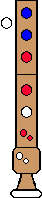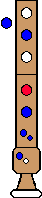Top

## Turned Trills

Turned Trill
Full step above, Full step below
Gb (alt) E D E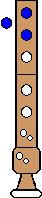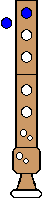Top

Turned Trill
Half step above; Full step below

(See below for alternate)
F E (alt) D EAlternate
Turned Trill
Half step above; Full step below
F E (alt) D EComments: Good when speed is necessary. You can also trill with all right hand fingers.
Top

Turned Trill
Full step above; Half step below
Gb (alt) E Eb E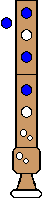Top

Turned Trill
Half step above; Half step below

(See below for alternate)
F E (alt) Eb EAlternate
Turned Trill
Half step above; Half step below
F E (alt) Eb (alt) E (alt)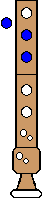Comments: Somewhat easier, but ends on alternate E
Top

## Regular Turns

Turn
Full step above; Full step below
Gb E D ETop

Turn
Half step above; Full step below
F E D ETop

Turn
Full step above; Half step below
Gb E Eb E